Question

What is the exercise value (intrinsic value) at the time of purchase (t = 0) to the holder of a put
with strike price \$120 if the underlying asset is \$125 and the put premium is \$5?
\$0
\$5
\$10

\$15
\$20

Intrinsic Value of Put Option = Max((Strike Price - Spot Price), 0)

So,

Intrinsic Value = Max((120 - 125), 0)

Intrinsic Value = \$0

#### Earn Coins

Coins can be redeemed for fabulous gifts.

Similar Homework Help Questions
• ### Suppose that you have taken a long position on a put option. The strike price is...

Suppose that you have taken a long position on a put option. The strike price is \$125, and the option premium / price is \$10. When the option expires, the value of the underlying asset is \$90. What is your pay-off and profit / loss?

• ### The intrinsic value of a put is equal to the: a) lesser of the stock price...

The intrinsic value of a put is equal to the: a) lesser of the stock price minus the exercise price or zero. b) greater of the strike price minus the stock price or zero. c) lesser of the stock price or zero. d) lesser of the strike price or the stock price. e) greater of the stock price minus the exercise price or zero.

• ### 2 points Q3. A put premium for a strike price of ¥4690 yen is ¥251 yen...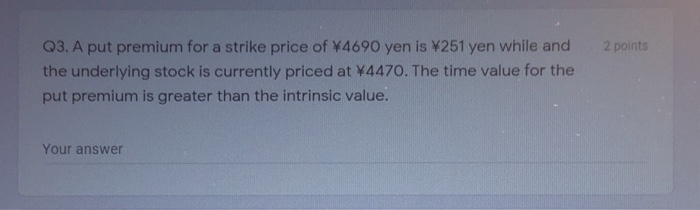2 points Q3. A put premium for a strike price of ¥4690 yen is ¥251 yen while and the underlying stock is currently priced at W4470. The time value for the put premium is greater than the intrinsic value. Your answer

• ### Suppose that you have taken a long position on a put option. The strike price is...

Suppose that you have taken a long position on a put option. The strike price is \$125, and the option premium / price is \$10. When the option expires, the value of the underlying asset is \$90. What is your pay-off and profit / loss? please show your work

• ### A stock is currently selling for \$75 per share. You could purchase a call with a...

A stock is currently selling for \$75 per share. You could purchase a call with a strike price of \$70 for \$7. You could purchase a put with a strike price of \$70 for \$2. Calculate the intrinsic value of the call option. Calculate the time value of the call option. Calculate the intrinsic value of the put option. Calculate the time value of the put option.

• ### It PusUNUI Cakcyl pom . 9) Consider the following example: SP = \$75, UA = \$95,...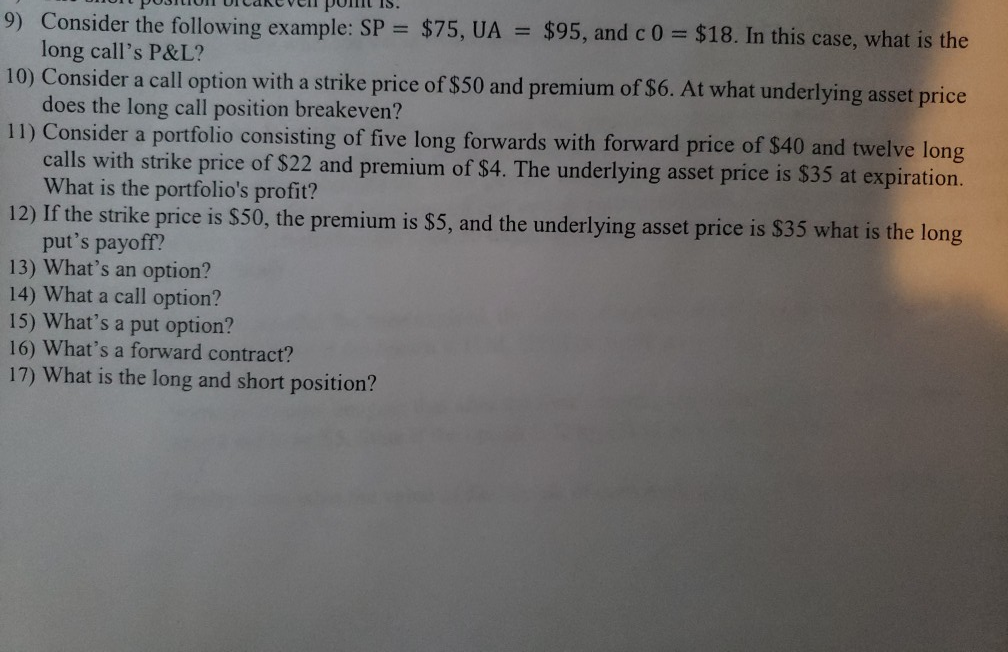It PusUNUI Cakcyl pom . 9) Consider the following example: SP = \$75, UA = \$95, and c 0 = \$18. In this case, what is the long call's P&L? 10) Consider a call option with a strike price of \$50 and premium of \$6. At what underlying asset price does the long call position breakeven? 11) Consider a portfolio consisting of five long forwards with forward price of \$40 and twelve long! calls with strike price of \$22 and...

• ### b. A March \$100,000 Treasury Bond put option with a strike price of 155-00 points has...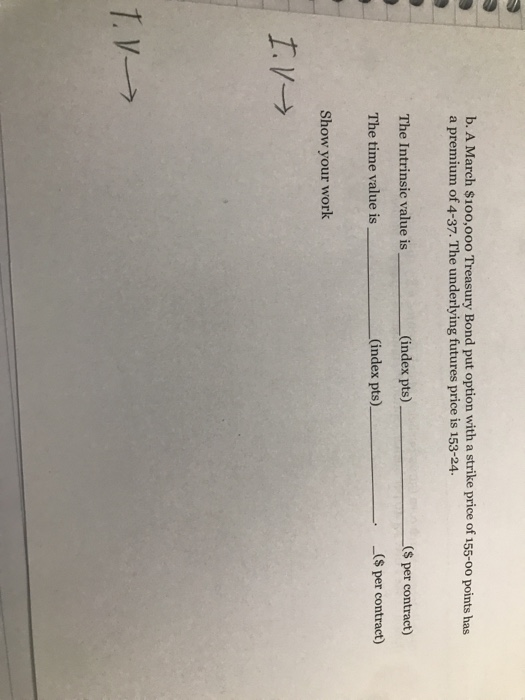b. A March \$100,000 Treasury Bond put option with a strike price of 155-00 points has a premium of 4-37. The underlying futures price is 153-24. The Intrinsic value is (index pts) (index pts) _(\$ per contract) _(\$ per contract) The time value is Show your work I. T.

• ### 2. Exercise value and option price The value derived from exercising an option immediately is the...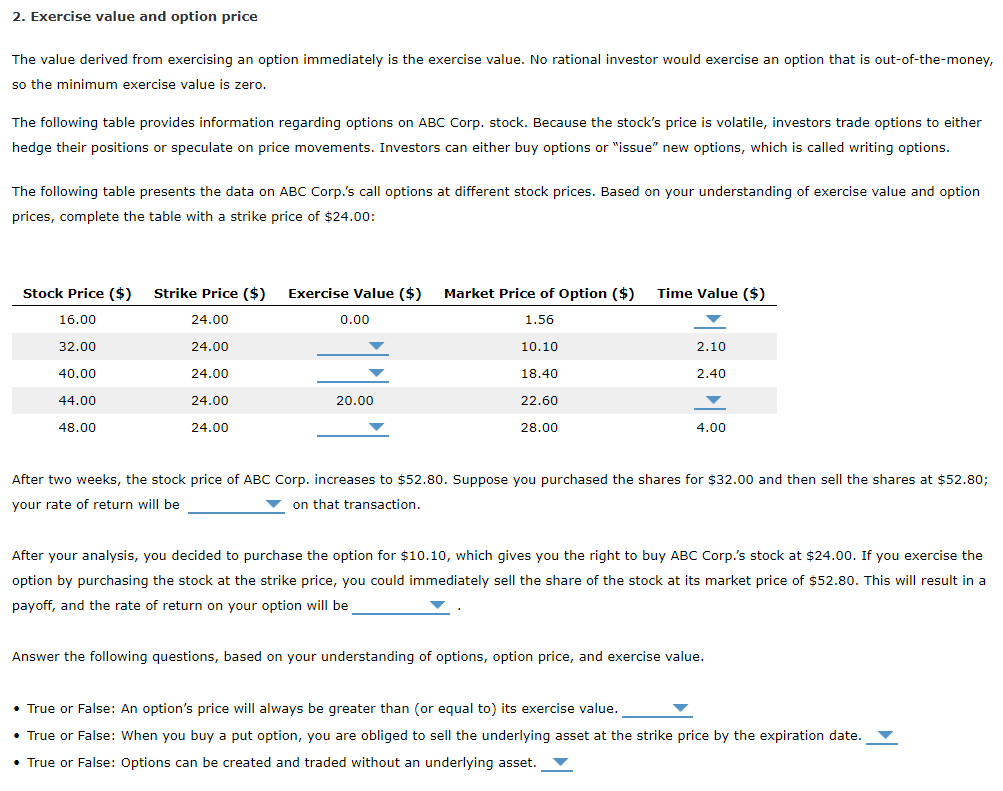2. Exercise value and option price The value derived from exercising an option immediately is the exercise value. No rational investor would exercise an option that is out-of-the-money, so the minimum exercise value is zero. The following table provides information regarding options on ABC Corp. stock. Because the stock's price is volatile, investors trade options to either hedge their positions or speculate on price movements. Investors can either buy options or "issue" new options, which is called writing options. The...

• ### Which of the following call options (X is the exercise price and T is time to...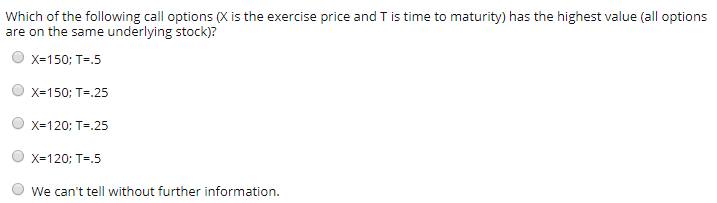Which of the following call options (X is the exercise price and T is time to maturity) has the highest value (all options are on the same underlying stock)? X=150; T=.5 X=150; T= 25 X=120; T=.25 X=120; T= 5 We can't tell without further information.

• ### A call option with a strike price of \$50 on a stock selling at 60 costs...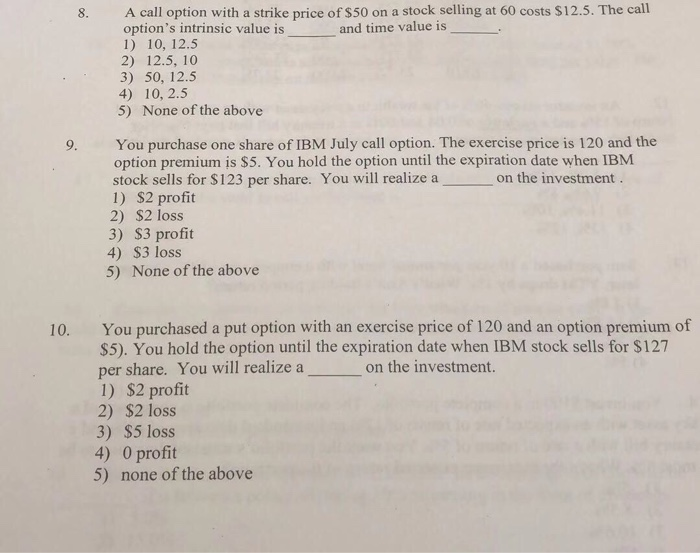A call option with a strike price of \$50 on a stock selling at 60 costs \$12.5. The call option's intrinsic value is 1) 10, 12.5 2) 12.5, 10 3) 50, 12.5 4) 10, 2.5 5) None of the above 8. and time value is You purchase one share of IBM July call option. The exercise price is 120 and the option premium is \$5. You hold the option until the expiration date when IBM stock sells for \$123 per...